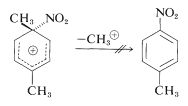# 22.7: IPSO Substitution

$$\newcommand{\vecs}{\overset { \rightharpoonup} {\mathbf{#1}} }$$ $$\newcommand{\vecd}{\overset{-\!-\!\rightharpoonup}{\vphantom{a}\smash {#1}}}$$$$\newcommand{\id}{\mathrm{id}}$$ $$\newcommand{\Span}{\mathrm{span}}$$ $$\newcommand{\kernel}{\mathrm{null}\,}$$ $$\newcommand{\range}{\mathrm{range}\,}$$ $$\newcommand{\RealPart}{\mathrm{Re}}$$ $$\newcommand{\ImaginaryPart}{\mathrm{Im}}$$ $$\newcommand{\Argument}{\mathrm{Arg}}$$ $$\newcommand{\norm}{\| #1 \|}$$ $$\newcommand{\inner}{\langle #1, #2 \rangle}$$ $$\newcommand{\Span}{\mathrm{span}}$$ $$\newcommand{\id}{\mathrm{id}}$$ $$\newcommand{\Span}{\mathrm{span}}$$ $$\newcommand{\kernel}{\mathrm{null}\,}$$ $$\newcommand{\range}{\mathrm{range}\,}$$ $$\newcommand{\RealPart}{\mathrm{Re}}$$ $$\newcommand{\ImaginaryPart}{\mathrm{Im}}$$ $$\newcommand{\Argument}{\mathrm{Arg}}$$ $$\newcommand{\norm}{\| #1 \|}$$ $$\newcommand{\inner}{\langle #1, #2 \rangle}$$ $$\newcommand{\Span}{\mathrm{span}}$$$$\newcommand{\AA}{\unicode[.8,0]{x212B}}$$

For all practical purposes, electrophilic aromatic substitution is confined to the substitution of a ring hydrogen. Does this mean that an electrophile such as $$\ce{NO_2^+}$$ only attacks hydrogen-bearing carbons? What about substituted ring carbons?

Electrophilic attack at methyl-bearing carbons, particularly in ortho- and para-dimethylbenzenes, would appear quite reasonable because the electron-donating character of the other methyl group should activate the ring by stabilizing the intermediate ion:Attack at the substituted (ipso) carbon evidently does occur, but it does not lead directly to substitution products because demethylation, unlike deprotonation, does not occur:Instead, the nitro group changes positions to the neighboring ring carbon, which then can eliminate a proton to form a substitution product:Because the product obtained indirectly (by ipso substitution) is indistinguishable from that expected by direct electrophilic attack at $$\ce{C_2}$$, it is not possible to say how much, if any, product is formed by the ipso route in this reaction.

In general, orientation effects in the substitution of alkylbenzenes are complicated by ipso attack. For example, in the nitration of 4-methylisopropylbenzene (para-cymene) about $$10\%$$ of the nitration product is 4-nitromethylbenzene:The 4-nitromethylbenzene arises from ipso attack of $$\ce{NO_2^+}$$ at the isopropyl-substituted ring carbon. Unlike methyl, the isopropyl group is eliminated rapidly as propene. Can we say that the other products, $$3$$ and $$4$$, arise by direct substitution? Evidently not, because nitration at $$0^\text{o}$$ gives two other products, $$5$$ and $$6$$, which must be formed by ipso attack at the methyl-bearing carbon. At low temperatures, intermediate ion $$7$$ is attacked by the weakly nucleophilic ethanoate ion to give $$5$$ and $$6$$. Both of these adducts solvolyze rapidly in $$78\%$$ sulfuric acid to give $$3$$ only: